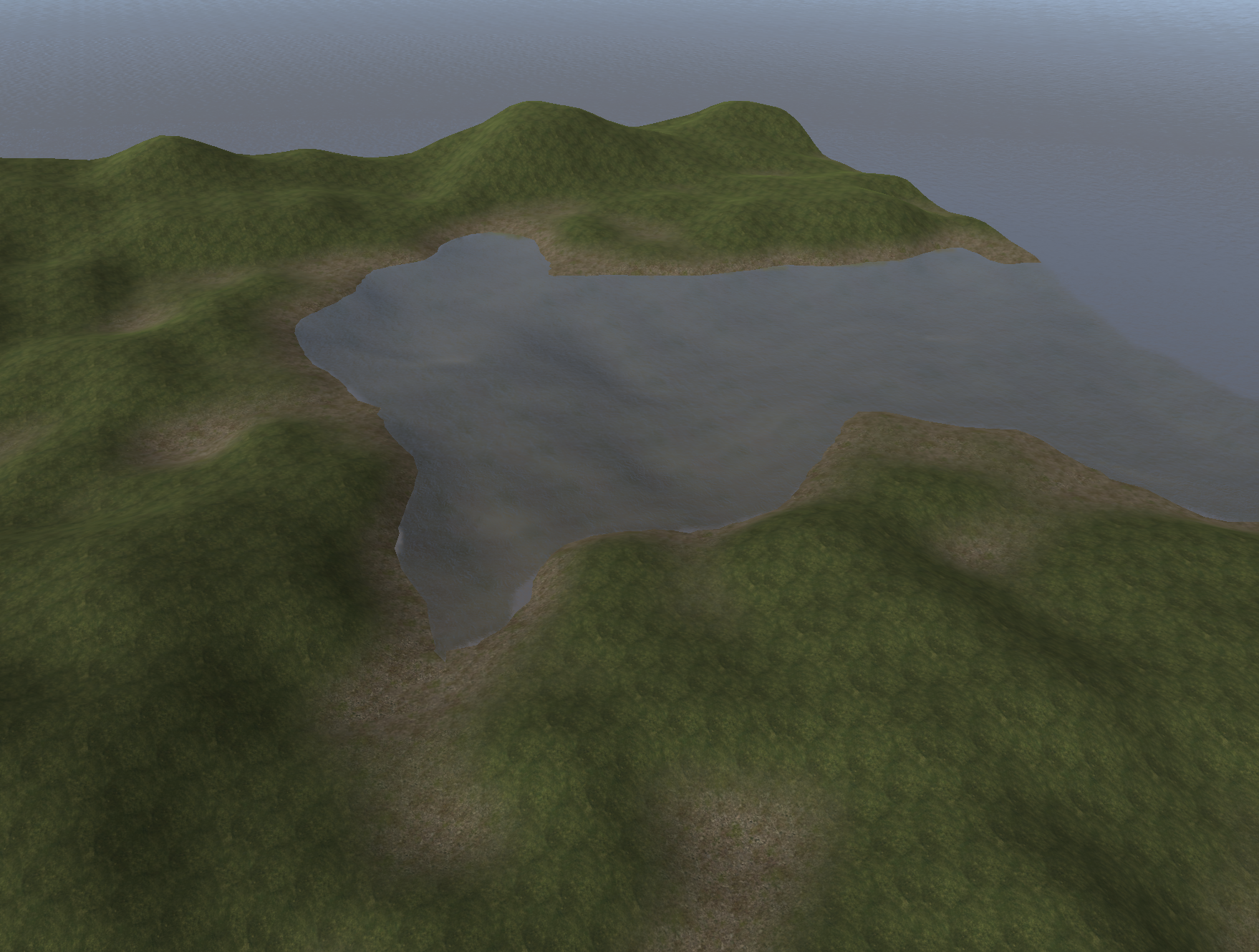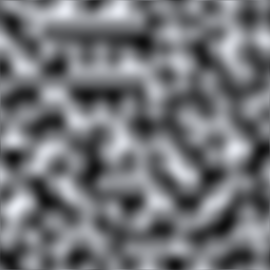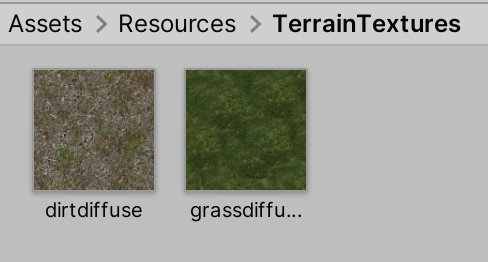# Building a world generation framework: Part 1Gonna write about my experiences and learnings implementing procedural world generation in my currently under development and untitled game. There are a ton of really helpful videos, tutorials and guides already out there on this topic of course, so this post is about tying it all together and implementing an entire world generation framework from scratch. Features of this framework include:

## First step: generating noise

In most procedurally generated game worlds out there, you are going to see some sort of gradient noise-based algorithm that creates the terrain. Perlin noise is a commonly found gradient noise used for this purpose. Gradient noise is nice because it randomly generates organic-looking rolling hills over an infinitely sized area. If you designate a certain height level as the water level, then you end up with a collection of islands, lakes, or small ponds, depending on how high you set the water.A Perlin noise example. The white parts represent values closer to 1, and the black parts represent values closer to 0. The resulting terrain will have hills in the white parts and valleys in the black parts source:https://docs.unity3d.com/ScriptReference/Mathf.PerlinNoise.html

Also beneficial with this kind of noise is the fact that generating the same “location” will always yield the same terrain. This is especially nice for games with infinite worlds where generating the whole world all at once isn’t feasible, so it has to be done on-demand, depending on the player’s location in the world for example. This is where “chunk”-based generation comes into play where the terrain is split up into discrete chunks that can be generated seamlessly as a player continues to explore the game world. A game that is definitely famous for this is Minecraft.

In Unity, you can use the `Mathf.PerlinNoise()` function to get some noise values given input x and y float coordinates:

```float height = Mathf.PerlinNoise(x, y);
```

The input coordinates can be any size, and the output will be a float between 0 and 1, representing how tall the terrain should be at that point.

Using this to generate a heightmap:

```float[,] generateHeightmap(int heightmapSize) {
float[,] heightmap = new float[heightmapSize, heightmapSize];

for (int i = 0; i < heightmapSize; ++i) {
for (int j = 0; j < heightmapSize; ++j) {
float xCoord = (float) i / heightmapSize;
float yCoord = (float) j / heightmapSize;

heightmap[i, j] = Mathf.PerlinNoise(xCoord, yCoord);
}
}

return heightmap;
}
```

Then assigning this to a Unity terrain:

```GameObject createTerrainGameObject() {
float maxTerrainHeight = 32f;
int heightmapSize = 129; // a power of two plus one

// Instantiate and set up terrain game object
GameObject terrainObj = new GameObject("Terrain");
terrainObj.transform.position = Vector3.zero;

Terrain terrain = terrainObj.AddComponent<Terrain>();
terrain.materialTemplate = new

TerrainCollider collider = terrainObj.AddComponent<TerrainCollider>();

// Create TerrainData with our generated heightmap
TerrainData terrainData = new TerrainData {
heightmapResolution = heightmapSize,
size = new Vector3(heightmapSize, maxTerrainHeight, heightmapSize)
};
terrainData.SetHeights(0, 0, generateHeightmap(heightmapSize));

terrain.terrainData = terrainData;
collider.terrainData = terrainData;

return terrainObj;
}
```

If you stick this into an `Awake()` in some component attached to a GameObject in your Unity scene, you will see a very simple terrain without any textures show up:

It will look the same every time you run it, since we’re not seeding it randomly, but that will come later.

## Extending the noise

Here’s a function called `basicNoise()` used in my game that extends the above Perlin noise with some extra functionality:

```float basicNoise(float xCoord, float yCoord, int octaves) {
float lacunarity = 2f;
float gain = 0.5f;

float sum = 0f;
float freq = 1f;
float amp = 1f;
float comp = 0f;

for (int i = 0; i < octaves; ++i) {
float res = Mathf.PerlinNoise(xCoord * freq, yCoord * freq);

sum += res * amp - comp;
freq *= lacunarity;
amp *= gain;
comp = amp / 2f;
}

return sum;
}
```

The function takes in x and y coordinates along with a number of `octaves`, which is how many times the noise should be “layered on” to create a more rougher look. I won’t go into the specifics of everything else in this function given that it’s already covered very well online. If you want to learn more about what all the various parts are and a better explanation about `octaves`, check out the following resources:

Modify `generateHeightmap()` to use this new noise function:

```...

for (int i = 0; i < heightmapSize; ++i) {
for (int j = 0; j < heightmapSize; ++j) {
float xCoord = (float) i / heightmapSize;
float yCoord = (float) j / heightmapSize;

heightmap[i, j] = basicNoise(xCoord, yCoord, 5);
}
}

...
```

Using the new noise function with 5 octaves makes the terrain look a bit more interesting:

## Texturing

That terrain looks kind of boring without any textures. Let’s update our framework to add some. To do this, we’ll need to add some texture layers and alphamap to the unity `TerrainData`. The alphamap is a 3d float array, indexed by x, y, and layer. So for any given point in the alphamap, it can have any number of layers with a 0-1 intensity. All layers should add up to 1 for a specific spot, otherwise you’ll get dark and bright spots where the values are less than 1 and greater than 1 respectively.

Let’s add grass and dirt. Here they are in Resources/TerrainTextures:Dirt and grass textures, named dirtdiffuse.tga and grassdiffuse.tga respectively

First create a function to generate an alphamap, in this case just setting everything to show the grass texture at 100%:

```const int GRASS_LAYER_INDEX = 0;
const int DIRT_LAYER_INDEX = 1;

...

float[,,] generateAlphamap(int alphamapSize) {
float[,,] alphamap = new float[alphamapSize, alphamapSize, 2];

for (int i = 0; i < alphamapSize; ++i) {
for (int j = 0; j < alphamapSize; ++j) {
alphamap[i, j, GRASS_LAYER_INDEX] = 1f;
}
}

return alphamap;
}
```

Update `createTerrainGameObject()` to use this new alphamap:

```GameObject createTerrainGameObject() {
float maxTerrainHeight = 32f;
int heightmapSize = 129; // a power of two plus one
int alphamapSize = 129; // a power of two plus one

// Instantiate and set up terrain game object
GameObject terrainObj = new GameObject("Terrain");

TerrainLayer[] terrainLayers = {
new TerrainLayer {
tileSize = new Vector2(5f, 5f),
},
new TerrainLayer {
tileSize = new Vector2(5f, 5f),
}
};

Terrain terrain = terrainObj.AddComponent<Terrain>();
terrain.materialTemplate = new

TerrainCollider collider = terrainObj.AddComponent<TerrainCollider>();

// Create TerrainData with our generated heightmap
TerrainData terrainData = new TerrainData {
heightmapResolution = heightmapSize,
alphamapResolution = alphamapSize,
terrainLayers = terrainLayers,
size = new Vector3(heightmapSize, maxTerrainHeight, heightmapSize)
};
terrainData.SetHeights(0, 0, generateHeightmap(heightmapSize));
terrainData.SetAlphamaps(0, 0, generateAlphamap(alphamapSize));

terrain.terrainData = terrainData;
collider.terrainData = terrainData;

return terrainObj;
}
```

Note that the terrain layer order is important here (lines 9-18). The first one we define is the grass texture, and the second one is the dirt texture, resulting in a 0 and 1 index in the alphamaps, respectively.

Now we get some grass:

## Water

Let’s add water and use the dirt texture added in the previous section for a nice transition between the grass and the waterline. You can use one of the nice looking water prefabs in Unity’s Standard Assets package.

Find WaterProDaytime.prefab:

Drag it into Assets/Resources so we’ll be able to instantiate it at runtime:

Update our code to add it to the scene:

```const float WATER_LEVEL = 10f;

void Awake() {
createTerrainGameObject();
createWaterObject(WATER_LEVEL);
}

...

GameObject createWaterObject(float waterLevel) {
GameObject waterObject = Instantiate(Resources.Load<GameObject>("WaterProDaytime"));
waterObject.transform.position = new Vector3(0f, waterLevel, 0f);
waterObject.transform.localScale = new Vector3(1000f, 1f, 1000f);

return waterObject;
}
```

Notice that the water is placed at y level 10, and that it’s scaled horizontally 1000×1000. The height ends up being in a good spot for the terrain we have generated so far (we’ll customize this later), and the scaling makes sure it extends out enough for now. For truly infinite worlds, we’ll of course need a better system for creating the water mesh further out.

Here’s our terrain with water:

## Height-based texturing

Let’s use the dirt texture we added early, and show it around the waterline. We’ll need to update the alphamap generating function to change the values depending on terrain height. To start, let’s make it so that everything below the (waterline + 0.5) is 100% dirt, a nice fade into grass over the next 1 unit of height, then 100% grass above that.

Since we’re factoring in terrain height, we need the alphamap generation function to take this into account. Modify the signature to take in the heightmap and max terrain height, as well as the waterline height:

```float[,,] generateAlphamap(int alphamapSize, float[,] heightmap, float maxTerrainHeight, float waterLevel) {
float[,,] alphamap = new float[alphamapSize, alphamapSize, 2];

for (int i = 0; i < alphamapSize; ++i) {
for (int j = 0; j < alphamapSize; ++j) {
float terrainHeight = heightmap[i, j] * maxTerrainHeight;

if (terrainHeight < waterLevel + 0.5f) {
// Dirt
alphamap[i, j, DIRT_LAYER_INDEX] = 1f;
} else if (terrainHeight > waterLevel + 0.5f && terrainHeight < waterLevel + 1.5f) {
float difference = waterLevel + 1.5f - terrainHeight;

// Dirt fading out upwards
alphamap[i, j, DIRT_LAYER_INDEX] = difference;
// Grass fading in upwards
alphamap[i, j, GRASS_LAYER_INDEX] = 1f - difference;
} else {
// Grass
alphamap[i, j, GRASS_LAYER_INDEX] = 1f;
}
}
}

return alphamap;
}
```

On line 6, we calculate the terrain height in world space by indexing the heightmap and multiplying the result (a float between 0 and 1) by the max terrain height. We can do this indexing because the alphamap and heightmap are the same size, but it’s easy enough to convert if they were different.

In the `else if` block between lines 11 and 18, we simply linearly interpolate the dirt and grass values based on height.

We’ll get something like this, looking a bit better than just solid grass:

The dirt shows up in areas not adjacent to the water, but that’s fine for now. We’ll explore more advanced terrain texture layer generation later on. Also it’s easy to see that we’ve just generated a “chunk” of terrain here. To create more terrain in the neighboring empty “spots” is fairly simple, we just offset the x and z positions for the terrain object by the chunk coordinates multiplied by the chunk width.

## Next steps

That’s it for Part 1. To recap, we’ve created a way to generate a terrain with some basic noise using Unity terrain/terrain data, texture it using terrain layers, add water, and update the texturing to take terrain height into account.

You can download a sample project containing all of the code above here:

In Part 2, we’ll refactor and expand the framework to handle multiple chunks based on a player’s movement, explore more complex and interesting noise for a more varied terrain, slope based texturing, and more.

Stay tuned.

Sergei Bezborodko

Founder of Original Studios